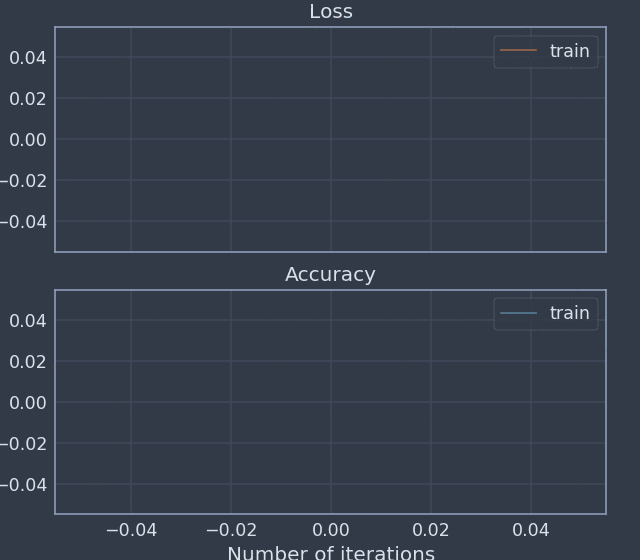# Noggin¶

Noggin is a simple Python tool for ‘live’ logging and plotting measurements during an experiment. Although Noggin can be used in a general context, it is designed around the train/test and batch/epoch paradigm for training a machine learning model.

Noggin’s primary features are its abilities to:

• Log batch-level and epoch-level measurements by name
• Seamlessly update a ‘live’ plot of your measurements, embedded within a Jupyter notebook
• Organize your measurements into a data set of arrays with labeled axes, via xarray

## A Simple Example of Using Your Noggin¶

Here is a sneak peak of what it looks like to use Noggin to record and plot data during an experiment. The following code is meant to be run in a Jupyter notebook.

```%matplotlib notebook
import numpy as np
from noggin import create_plot
metrics = ["accuracy", "loss"]
plotter, fig, ax = create_plot(metrics)

for i, x in enumerate(np.linspace(0, 10, 100)):
# record and plot batch-level metrics
x += np.random.rand(1)*5
batch_metrics = {"accuracy": x**2, "loss": 1/x**.5}
plotter.set_train_batch(batch_metrics, batch_size=1, plot=True)

# record training epoch
if i%10 == 0 and i > 0:
plotter.set_train_epoch()

# cue test-evaluation of model
for x in np.linspace(0, 10, 5):
x += (np.random.rand(1) - 0.5)*5
test_metrics = {"accuracy": x**2}
plotter.set_test_batch(test_metrics, batch_size=1)
plotter.set_test_epoch()
plotter.plot()  # ensures final data gets plotted
```